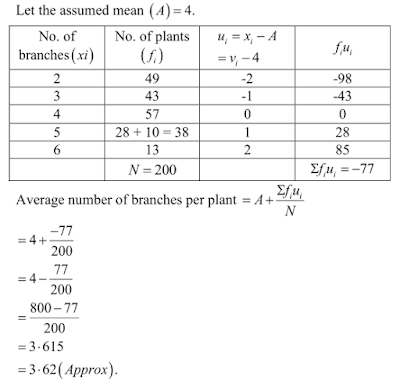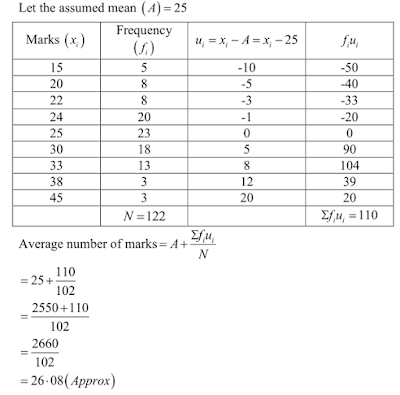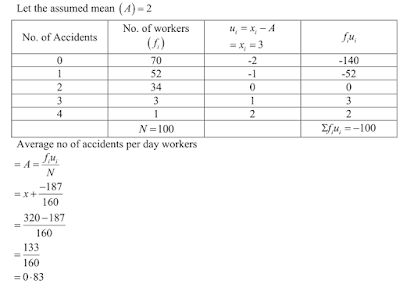## Chapter 7 Statistics R.D. Sharma Solutions for Class 10th Math Exercise 7.2

1. The number of telephone calls received at an exchange per interval for 250 successive one- minute intervals are given in the following frequency table:
No. of calls(x): 0 1 2 3 4 5 6
No. of intervals (f): 15 24 29 46 54 43 39
Compute the mean number of calls per interval.

Solution2. Five coins were simultaneously tossed 1000 times, and at each toss the number of heads was observed. The number of tosses during which 0,1,2,3,4 and 5 heads were obtained are shown in the table below. Find the mean number of heads per toss
No. of heads per toss (x): 0     1    2    3      4     5
No. of tosses (f):              38 144 342 287 164 25

Solution3. The following table gives the number of branches and number of plants in the garden of a
school.
No. of branches (x): 2   3   4   5   6
No. of plants     (f): 49 43 57 38 13
Calculate the average number of branches per plant.

Solution4. The following table gives the number of children of 150 families in a village
No. of children (x): 0 1 2 3 4 5
No. of families (f): 10 21 55 42 15 7
Find the average number of children per family.

Solution5. The marks obtained out of 50, by 102 students in a Physics test are given in the frequency

table below:
Marks(x):       15 20 22 24 25 30 33 38 45
Frequency (f): 5  8   11 20 23 18 13  3  1
Find the average number of marks.

Solution6. The number of students absent in a class were recorded every day for 120 days and the information is given in the following frequency table:
No. of students absent (x): 0 1   2   3   4   5 6  7
No. of days (f):                   1 4 10 50 34 15 4 2
Find the mean number of students absent per day.

Solution7. In the first proof reading of a book containing 300 pages the following distribution of
misprints was obtained:
No. of misprints per page (x): 0     1     2    3   4   5
No. of pages (f):                      154 95  36   9   5   1
Find the average number of misprints per page.

Solution8. The following distribution gives the number of accidents met by 160 workers in a factory
during a month.
No. of accidents (x): 0 1 2 3 4
No. of workers (f): 70 52 34 3 1
Find the average number of accidents per worker.

Solution9. Find the mean from the following frequency distribution of marks at a test in statistics:
Marks(x): 5 10 15 20 25 30 35 40 45 50
No. of students (f): 15 50 80 76 72 45 39 9 8 6

Solution